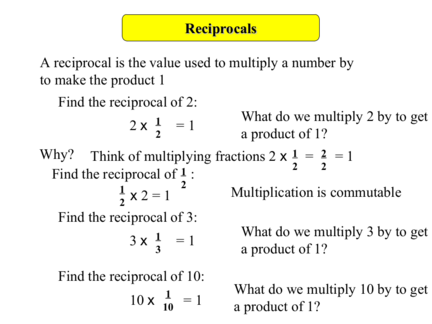# Finding Reciprocals of Fractions and Mixed NumbersContributed by:This pdf includes:-
Reciprocal
How to find reciprocal
What is the use of reciprocal
1. Reciprocals
A reciprocal is the value used to multiply a number by
to make the product 1
Find the reciprocal of 2:
1 What do we multiply 2 by to get
2x =1
2 a product of 1?
Why? Think of multiplying fractions 2 x 1 = 2 = 1
1 2 2
Find the reciprocal of :
1 2
x 2 = 1 Multiplication is commutable
2
Find the reciprocal of 3:
1 What do we multiply 3 by to get
3x =1
3 a product of 1?
Find the reciprocal of 10:
1 What do we multiply 10 by to get
10 x 10
=1 a product of 1?
2. Reciprocals
3
Find the reciprocal of 4
:
3 x 4 =1 What do we multiply 3 by to get
4 3 4
a product of 1?
Remember how to multiply fractions: 3 x 4 = 12 =1
4 3 12
Find the reciprocal of 0.7 :
7 x 10 = 1
0.7 = 7
10 10 7
10 3
Now change into a mixed number 17
7
So the reciprocal of 0.7 is 13
7
3. Reciprocals
Find the reciprocal of 2 2
3
First write the mixed number as a top heavy fraction
8 3
then follow the same process 8
3
Now you know the principles the shortcut to finding a reciprocal
is to swap the numerator and denominator over
8 x =1
3
4. Reciprocals
Now do these
Find the reciprocals of:
1. 6
1
7.
3 3 23
6 11
2. 7 1
8. 0.3 3 13
7
3. 0.25 4 9. 0.125 8
1 3 4
4. 8 10. 1 7
8 4
1 4
5. 12 11. 1.25 5
12
2 1
. 3
6. 3 12. 1.6 5
7 2